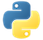# CountPydoc

Counts the number of elements within each aggregation.

## Examples

In the following example, we create a pipeline with two `PCollection`s of produce. Then, we apply `Count` to get the total number of elements in different ways.

### Example 1: Counting all elements in a PCollection

We use `Count.Globally()` to count all elements in a `PCollection`, even if there are duplicate elements.

``````import apache_beam as beam

with beam.Pipeline() as pipeline:
total_elements = (
pipeline
| 'Create plants' >> beam.Create(
['🍓', '🥕', '🥕', '🥕', '🍆', '🍆', '🍅', '🍅', '🍅', '🌽'])
| 'Count all elements' >> beam.combiners.Count.Globally()
| beam.Map(print))``````

Output:

``10``View source code

### Example 2: Counting elements for each key

We use `Count.PerKey()` to count the elements for each unique key in a `PCollection` of key-values.

``````import apache_beam as beam

with beam.Pipeline() as pipeline:
total_elements_per_keys = (
pipeline
| 'Create plants' >> beam.Create([
('spring', '🍓'),
('spring', '🥕'),
('summer', '🥕'),
('fall', '🥕'),
('spring', '🍆'),
('winter', '🍆'),
('spring', '🍅'),
('summer', '🍅'),
('fall', '🍅'),
('summer', '🌽'),
])
| 'Count elements per key' >> beam.combiners.Count.PerKey()
| beam.Map(print))``````

Output:

``````('spring', 4)
('summer', 3)
('fall', 2)
('winter', 1)``````View source code

### Example 3: Counting all unique elements

We use `Count.PerElement()` to count the only the unique elements in a `PCollection`.

``````import apache_beam as beam

with beam.Pipeline() as pipeline:
total_unique_elements = (
pipeline
| 'Create produce' >> beam.Create(
['🍓', '🥕', '🥕', '🥕', '🍆', '🍆', '🍅', '🍅', '🍅', '🌽'])
| 'Count unique elements' >> beam.combiners.Count.PerElement()
| beam.Map(print))``````

Output:

``````('🍓', 1)
('🥕', 3)
('🍆', 2)
('🍅', 3)
('🌽', 1)``````View source code

N/APydoc